## 9 September 2013

### Working with geometric objects

In this page we will go through step-by-step solutions for some of the geometric object type of questions commonly asked in CEED exams. These type of pictorial questions were asked in exams to test your visual ability skills and you are required to solve these questions without the help of paper or pen so as to save time.

<< back to CEED resource page

#### Question : 1

Count the number of faces on geometric objects

This type of visualization can be solved by counting number of faces on each direction. You can view the solid in six different directions. They are front-right-back-left-top-bottom. Fix one particular direction/order to eliminate confusion. Now you can see the faces in the below images. The object face that can be directly seen has been shaded (hatched) in blue color while the face which is hidden has been hatched in red color for your convenience.

Total number of faces is thus 16

#### Question : 2

Correct reflection of composition by reflecting four times along the edges.

For this type of questions, you need to fix the direction of mirroring (for this example it is clock-wise). Firstly consider only the main object and first reflection image as shown in step-1 below; forget about the remaining part.

Next consider only the first reflected image and the next reflection to be carried and forget about the remaining triangles. Like this continue visually and thus you will come to the final solution. You should try this mentally without using pen or paper.

#### Question : 3

For this type of questions, you need to identify the direction of the sun/source; in this case its from left to right. For this cube you will count eight sharp edges. Trick is "higher the edge from ground and farther the edge from the sun/source, farther will be the shadow and only the top and side edges will have visible shadows".

#### Question : 4

Count the number of small cubes missing in this figure

The best thing is to count the missing cubes row-rise, starting from bottom; without being confused. Here I have given number of row-wise missing cubes from the bottom.

Row 1 - 2 cubes missing
Row 2 - 1 cube missing
Row 3 - 3 cubes missing
Row 4 - 9 cubes missing ((4*4 - 7) = 16-7 = 9)

Like Stuff You Look blog on Facebook page to get connected with latest posts.

1.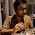Dude that ques3 U don't have to think about, the sun, or count edges

The question is simply about shape of shadow, U only have to think what is a shape of cubes shadow, cylinder or sphere just that

N thumpz up for ur blog, lots of data

1.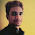ya, but u missed one imp thing, depending upon the direction of sun, ur shadow varies! so, it is always not the case of simply depending on usual shadow of the shapes!

2.Agree with Bhanu

2.Cool buddy, ur blog is really great, its evident U put a lot of time n effort

3.4.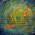bhanu sir ,in this question paper there is one question ( Q 19) based on cube missing pattern . when i did this i got 60 answer and but when i checked the answer key-
i found that there are 158 cube missing. i dont know where i m lacking. can u please check and provide me the solution.
thanks

1.did u tried the procedure that I mentioned above ? anyway I'm already making a post of UCEED'15 solutions. Will share soon, you can clear then.

2.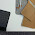Actually the answer was 58... soo think there was a printing mistake to 158..!

3.Hi Shivani, and Hi Bhanu Sir...
I think you misread the question.. it was to find the "no. of cubes".. not the number of cubes "missing"... + You seem to have made a mistake in the counting part too.. as Loga srinivasan said the number of missing cubes is 58...
So now, assuming that there were no cubes missing in the figure mentioned, the total number of cubes is 6x6x6 = 216... And you know there are 58 cubes missing...
So therefore the number of cubes remaining is just 216-58 = 158...
There you go... :D

5.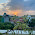Sir,
Would'nt there be 8 cubes missing in the 4th row for the 4th question?
Thanks

1.Yes Umang, that's 8, thanks you :)

2.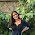I feel like there are 9 :P

3.in the 4th question....there are nine missing right???...how 8??

4.sorry guys, Umang comment confuse me :P, ya it's actually 9, I gave the explanation above. Thank u all

6.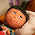gr8 blog man (Y)

7.Sir I couldn't get the meaning of question 2. Please explain only the meaning of question 2, so that I get what the question wants us to do. Thank you.

1.Given the image of the first (single) triangle with the (two) marks in it, if you mirror it several times (in this case 4 times); each time w.r.t an edge of the triangle as shown in successive triangles, where will be those marks appear in the fourth triangle, after four mirroring positions as shown in the images. I think you now understood the question.

8.Done sir. It was easy. Thank you.

9.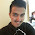What is the answer to Q4? 15,right?

1.10.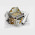Nice bro .u r doing Great job .Putting lots of efforts and spending lot of time for our need .Really hats off to u bhai

11.Great job sir!!

12.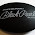in ques 2 , the inner square has its base parallel to the base of the larger triangle , but when it is mirrored how is the base of the square parallel to another side ? please explain.

13.can you pls post a pdf on counting the surface type question

14.